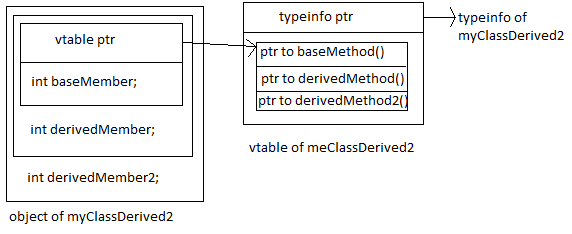# Virtual Function and Multilevel Inheritance

Consider these C++ classes
```class myClassBase
{
public:
int baseMember;
myClassBase() { baseMember = 0; }
virtual int baseMethod(int x) { return baseMember + x; }
};
class myClassDerived : public myClassBase
{
public:
int derivedMember;
myClassDerived() { derivedMember = 0; }
virtual int derivedMethod(int x) { return derivedMember + x; }
};
class myClassDerived2 : public myClassDerived
{
int derivedMember2;
virtual int derivedMethod2(int x) { return derivedMember2 + x; }
};

```
If we instantiate myClassDerived2 then
• The vtable pointer of the object will point to the vtable of myClassDerived2.
• There will be only one vtable pointer in the object. This is the one which is created by myClassBase.
• The typeinfo and vtable will have similar structure that we discussed in the previous examples.
• The vtable will have function pointers in the sequence of class hierarchy. It will first have the function pointers declared by myClassBase then of myClassDerived and then myClassDerived2. This sequence helps in resolving the function from all classes. For example if the function from the myClassBase (object pointer is of type myClassBase*) is being resolved, then it will consider that the vtable is of its own then use particular index for its function.
• If the derived class does not override any virtual function, then its vtable will have pointers to the functions of its base.

Here is the diagram describing the relations: